# Introduction

• 人脸检测。人脸检测器用于寻找图像中人脸的位置，如果有人脸，就返回包含每张人脸的边界框的坐标。如图 3a 所示。
• 人脸对齐。人脸对齐的目标是使用一组位于图像中固定位置的参考点来缩放和裁剪人脸图像。这个过程通常需要使用一个特征点检测器来寻找一组人脸特征点，在简单的 2D 对齐情况中，即为寻找最适合参考点的最佳仿射变换。图 3b 和 3c 展示了两张使用了同一组参考点对齐后的人脸图像。更复杂的 3D 对齐算法（如 ）还能实现人脸正面化，即将人脸的姿势调整到正面向前。
• 人脸表征。在人脸表征阶段，人脸图像的像素值会被转换成紧凑且可判别的特征向量，这也被称为模板（template）。理想情况下，同一个主体的所有人脸都应该映射到相似的特征向量。
• 人脸匹配。在人脸匹配构建模块中，两个模板会进行比较，从而得到一个相似度分数，该分数给出了两者属于同一个主体的可能性。• close-set：就是所有的测试集都在训练集中出现过。所以预测结果是图片的ID，如果想要测试两张图片是否是同一个，那么就看这两张图片的预测ID是否一样即可。
• open-set：就是测试的图片并没有在训练集中出现过，那么每张测试图片的预测结果是特征向量，如果想要比较两张图片的人脸是否属于同一个人，需要测试图像特征向量的距离。

1. 工程角度：研发质量模型，对检测到人脸质量进行评价，质量较差则不识别/检验。

2. 应用角度：施加场景限制，比如刷脸解锁，人脸闸机，会场签到时，都要求用户在良好的光照条件下正对摄像头，以避免采集到质量差的图片。

3. 算法角度：提升人脸识别模型性能，在训练数据里添加更多复杂场景和质量的照片，以增强模型的抗干扰能力。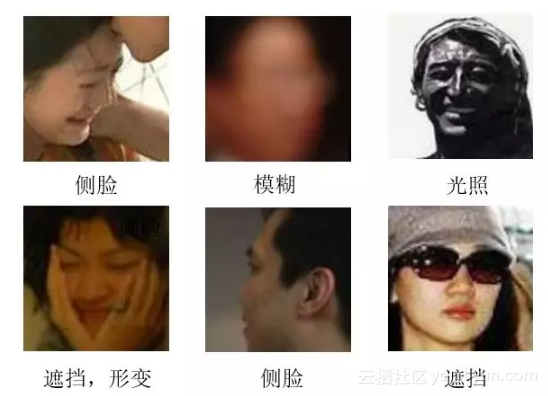# Euclidean Based Metric Learning

## Contrastive loss

DeepID1并不属于contrastive loss系列，只是作为DeepID系列的开山之作而放在这里。

DeepID1:《Deep Learning Face Representation from Predicting 10,000 Classes》

DeepID2:《Deep learning face representation by joint identification-verification》

DeepID2+:《Deeply learned face representations are sparse, selective, and robust》

DeepID3:《Deepid3: Face recognition with very deep neural networks》

$\operatorname{Ident}\left(f, t, \theta_{i d}\right)=-\sum_{i=1}^{n} p_{i} \log \hat{p}_{i}=-\log \hat{p}_{t}$

$\operatorname{Verif}\left(f_{i}, f_{j}, y_{i j}, \theta_{v e}\right)=\left\{\begin{array}{ll}{\frac{1}{2}\left\|f_{i}-f_{j}\right\|_{2}^{2}} & {\text { if } y_{i j}=1} \\ {\frac{1}{2} \max \left(0, m-\left\|f_{i}-f_{j}\right\|_{2}\right)^{2}} & {\text { if } y_{i j}=-1}\end{array}\right.$

DeepID1: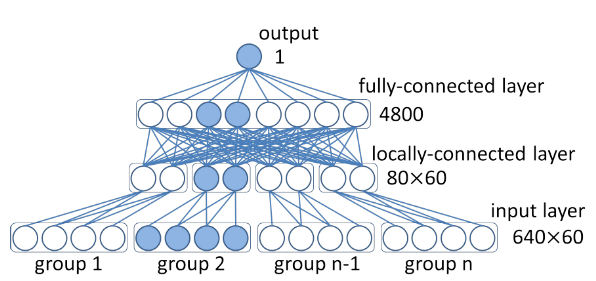DeepID2: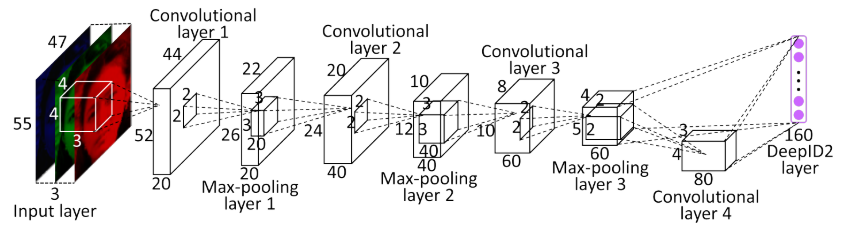DeepID2+: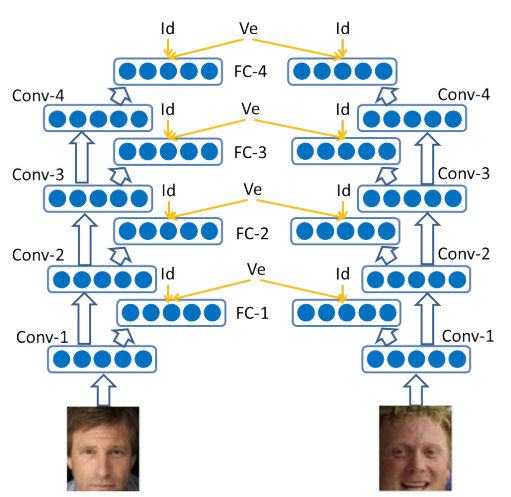DeepID3:## Triplet Loss

《Facenet: A unified embedding for face recognition and clustering》

$\sum_{i}^{N}\left[\left\|f\left(x_{i}^{a}\right)-f\left(x_{i}^{p}\right)\right\|_{2}^{2}-\left\|f\left(x_{i}^{a}\right)-f\left(x_{i}^{n}\right)\right\|_{2}^{2}+\alpha\right]$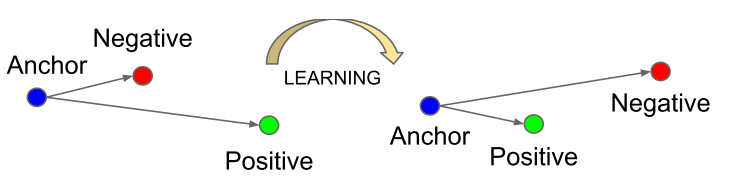## Center Loss

《A Discriminative Feature Learning Approach for Deep Face Recognition》

\begin{aligned} \mathcal{L} &=\mathcal{L}_{S}+\lambda \mathcal{L}_{C} \\ &=-\sum_{i=1}^{m} \log \frac{e^{W_{y_{i}}^{T} \boldsymbol{x}_{i}+b_{y_{i}}}}{\sum_{j=1}^{n} e^{W_{j}^{T} \boldsymbol{x}_{i}+b_{j}}}+\frac{\lambda}{2} \sum_{i=1}^{m}\left\|\boldsymbol{x}_{i}-\boldsymbol{c}_{y_{i}}\right\|_{2}^{2} \end{aligned}

$c_{y_i}$代表样本$i$对应类别$y_i$所属的类中心。理想情况下，这个类中心在每次迭代时都需要利用整个数据集的特征来更新，但是这会需要巨大的计算量。因此，在实际使用中，做了如下修改：

• 由整个训练集更新center改为mini-batch更新center；
• 避免错误分类的样本的干扰，使用scalar α 来控制center的学习率；## Center-Invariant Loss

《Deep face recognition with center invariant loss》

\begin{aligned} L=& L_{s}+\gamma L_{I}+\lambda L_{c} \\=&-\log \left(\frac{e^{\mathbf{w}_{y}^{T} \mathbf{x}_{i}+b_{y}}}{\sum_{j=1}^{m} e^{\mathbf{w}_{j}^{T} \mathbf{x}_{i}+b_{j}}}\right)+\frac{\gamma}{4}\left(\left\|\mathbf{c}_{y}\right\|_{2}^{2}-\frac{1}{m} \sum_{k=1}^{m}\left\|\mathbf{c}_{k}\right\|_{2}^{2}\right)^{2} \\ &+\frac{\lambda}{2}\left\|\mathbf{x}_{i}-\mathbf{c}_{y}\right\|^{2} \end{aligned}## Range Loss

《Range loss for deep face recognition with long-tail》

$\mathcal{L}_{R}=\alpha \mathcal{L}_{R_{i n t r a}}+\beta \mathcal{L}_{R_{i n t e r}}$

$\mathcal{L}_{R_{i n t r a}}=\sum_{i \subseteq I} \mathcal{L}_{R_{i n t r a}}^{i}=\sum_{i \subseteq I} \frac{k}{\sum_{j=1}^{k} \frac{1}{\mathcal{D}_{j}}}$

\begin{aligned} \mathcal{L}_{R_{\text { inter }}} &=\max \left(m-\mathcal{D}_{\text {Center}}, 0\right) \\ &=\max \left(m-\left\|\overline{x}_{Q}-\overline{x}_{\mathcal{R}}\right\|_{2}^{2}, 0\right) \end{aligned}

$\mathcal{L}=\mathcal{L}_{M}+\lambda \mathcal{L}_{R}=-\sum_{i=1}^{M} \log \frac{e^{W_{y_{i}}^{T} x_{i}+b_{v_{i}}}}{\sum_{j=1}^{n} e^{W_{j}^{T} x_{i}+b_{j}}}+\lambda \mathcal{L}_{R}$

## Summary

• 模型需要很长时间才能拟合，contrastive loss和triplet loss的训练样本都基于pair或者triplet的，可能的样本数是O(N2)或者O(N3)的。当训练集很大时，基本不可能遍历到所有可能的样本，所以一般来说需要很长时间才能拟合；
• 模型好坏很依赖训练数据的sample方式，理想的sample方式不仅能提升算法最后的性能，更能略微加快训练速度。

# Margin Based Classification

## Softmax Loss

$L=\frac{1}{N} \sum_{i} L_{i}=\frac{1}{N} \sum_{i}-\log \left(\frac{e^{f_{y_{i}}}}{\sum_{j} e^{f_{j}}}\right)$

$L_{i}=-\log \left(\frac{e^{\left\|\mathbf{W}_{y_{i}}\right\|\left\|\mathbf{x}_{i}\right\| \cos \left(\theta_{y_{i}}\right)}}{\sum_{j} e^{\left\|\mathbf{W}_{j}\right\|\left\|\mathbf{x}_{\mathbf{i}}\right\| \cos \left(\theta_{j}\right)})}\right)$

## L-Softmax Loss

《Large-margin softmax loss for convolutional neural networks》

$L_{i}=-\log \left(\frac{e^{\left\|\mathbf{W}_{y_{i}}\right\|\left\|\mathbf{x}_{i}\right\| \psi\left(\theta_{y_{i}}\right)}}{\left\|\mathbf{W}_{y_{i}}\right\|\left\|\mathbf{x}_{i}\right\| \psi\left(\theta_{y_{i}}\right)+\sum_{j \neq y_{i}} e^{\left\|\mathbf{W}_{j}\right\|\left\|\mathbf{x}_{i}\right\| \cos \left(\theta_{j}\right)})}\right)$

$\psi(\theta)=\left\{\begin{array}{l}{\cos (m \theta), 0 \leq \theta \leq \frac{\pi}{m}} \\ {\mathcal{D}(\theta), \frac{\pi}{m}<\theta \leq \pi}\end{array}\right.$

$\psi(\theta)=(-1)^{k} \cos (m \theta)-2 k, \theta \in\left[\frac{k \pi}{m}, \frac{(k+1) \pi}{m}\right]$

Large-Margin Softmax Loss是Softmax Loss引入margin思想的改进版本。即要是数据$X_i$不仅能够正确分类，而且与正确类别对应基向量的夹角要大于不同类别基向量的夹角一定的margin角度。因此在学习同类样本时，增强了同类学习的难度，这个难度要比不同类的难度要大些。这样的区别对待使得特征的可区分性增强。## A-Softmax Loss

《Sphereface: Deep hypersphere embedding for face recognition》

$L_{a n g}=\frac{1}{N} \sum_{i}-\log \left(\frac{\exp \left(\left\|x_{i}\right\| \psi\left(\theta_{y i, i}\right)\right)}{\exp \left(\left\|x_{i}\right\| \psi\left(\theta_{y i}, i\right)\right)+\sum_{j \neq y_{i}} \exp \left(\left\|x_{i}\right\| \cos \left(\theta_{j, i}\right)\right)}\right)$

A-Softmax与L-Softmax的最大区别在于A-Softmax的权重归一化了，而L-Softmax则没有。A-Softmax权重的归一化导致特征上的点映射到单位超球面上，而L-Softmax则不没有这个限制，这个特性使得两者在几何的解释上是不一样的。如图1所示，如果在训练时两个类别的特征输入在同一个区域时，如下图10所示。A-Softmax只能从角度上分度这两个类别，也就是说它仅从方向上区分类，分类的结果如图2所示；而L-Softmax，不仅可以从角度上区别两个类，还能从权重的模（长度）上区别这两个类，分类的结果如图3所示。在数据集合大小固定的条件下，L-Softmax能有两个方法分类，训练可能没有使得它在角度与长度方向都分离，导致它的精确可能不如A-Softmax。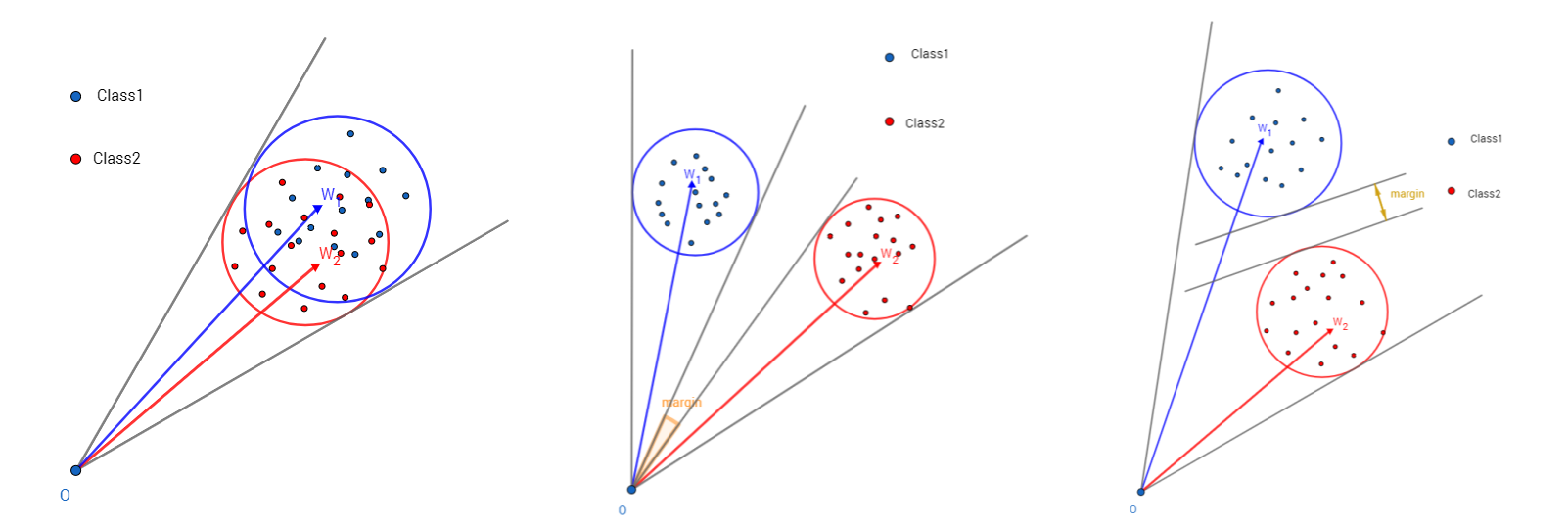A-Softmax虽然效果很好，但是存在着显著的问题。在测试阶段，A-Softmax通过特征间的余弦值来衡量相似性，即以角度为相似性的度量；但在训练阶段，A-Softmax并不是直接优化特征与类中心的角度，而是优化特征与类中心的角度再乘上一个特征的长度。因此，优化的方向还有一部分是去增大特征的长度。下图是A-Softmax在Mnist数据集上的实验，分别对应m=1和m=4时的特征可视化。注意坐标的尺度，就能验证上述观点。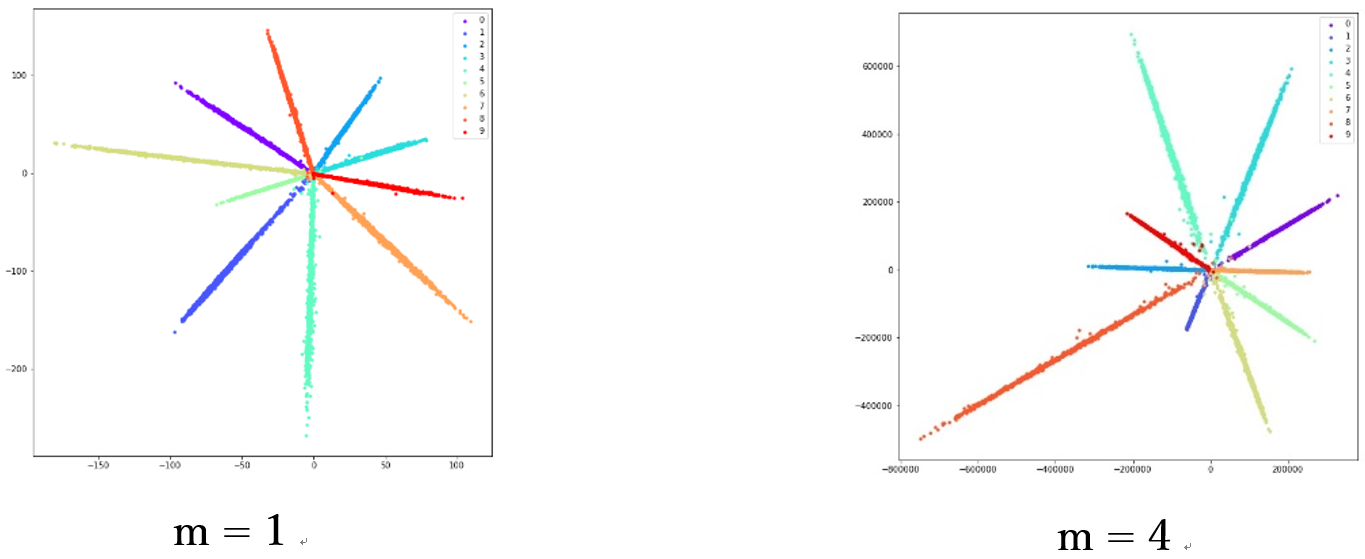## NormFace

NormFace: $L_2$ Hypersphere Embedding for Face Verification Feng

$\mathcal{L}_{\mathcal{S},}=-\frac{1}{m} \sum_{i=1}^{m} \log \frac{e^{s \tilde{W}_{y_{i}}^{T} \tilde{\mathrm{f}}_{i}}}{\sum_{j=1}^{n} e^{s \tilde{W}_{j}^{T} \tilde{\mathrm{f}}_{i}}}$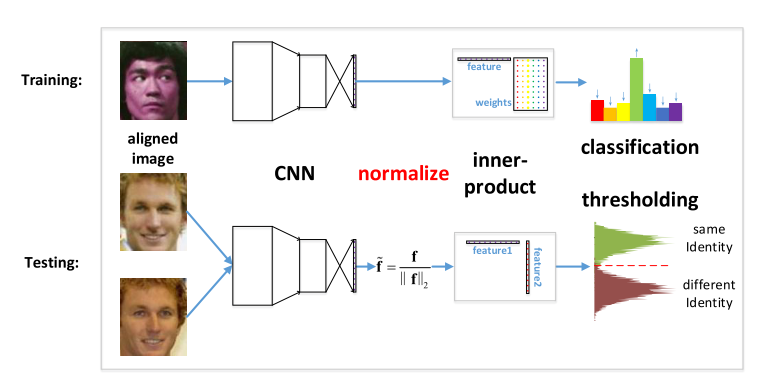## AM-Softmax Loss

《Additive Margin Softmax for Face Verification 》

$L_{A M S}=-\frac{1}{n} \sum_{i=1}^{n} \log \frac{e^{s \cdot\left(\cos \theta_{y_{i}}-m\right)}}{e^{s\left(\cos \theta_{y_{i}}-m\right)}+\sum_{j=1, j \neq y_{i}}^{c} e^{s \cdot \cos \left(\theta_{j}\right)}}$

AM-Softmax令$||x||=1, ||W||=1, b=0$$s$为缩放因子，论文里固定为30。

A-Softmax中为$\cos m\theta_{y_{i}}$，而AM-Softmax为$\cos\theta_{y_{i}}-m$。一个是角度距离（angular margin），一个是余弦距离（cosine margin）。当使用传统的softmax作为损失函数时，角度距离和余弦距离是等价的，即：$\cos \left(\theta_{1}\right)=\cos \left(\theta_{2}\right) \Rightarrow \theta_{1}=\theta_{2}$。但当试图改变决策边界时，角度距离和余弦距离便不再等价。最终的决策边界是和余弦相关的，根据cos的性质，优化角度距离比优化余弦距离更有效果，因为余弦距离相对更密集。（为什么论文采用优化余弦距离？）

AM-Softmax的好处在于A-Softmax的倍角计算是要通过倍角公式，反向传播时不方便求导，而只减m反向传播时导数不用变化。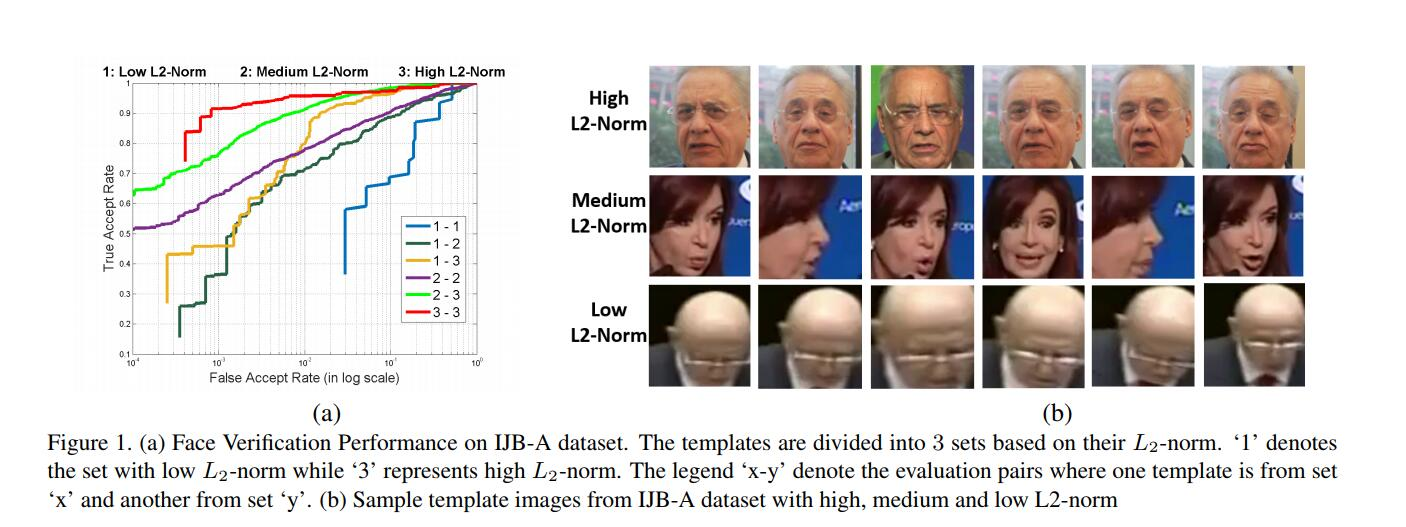Softmax的目标是尽可能最大化正确分类的概率。它会忽略掉一些比较难分辨的图片（低质量的图片），优先拟合高质量的图片。而L2-Softmax中提到，质量较差的人脸图片的特征范数越小。在进行了特征归一化后，这些质量较差的图片特征会产生更大的梯度，导致网络在训练过程中将更多的注意力集中在这些样本上。因此，对于数据集图片质量较差时，更适合采用特征归一化。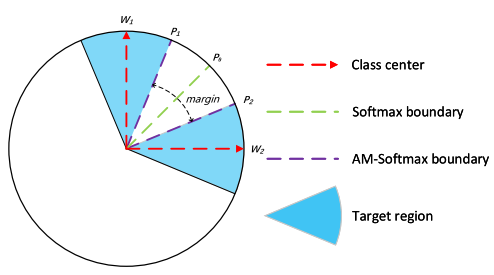## CosFace

CosFace: Large Margin Cosine Loss for Deep Face Recognition

$L_{l m c}=\frac{1}{N} \sum_{i}-\log \frac{e^{s\left(\cos \left(\theta_{y_{i}, i}\right)-m\right)}}{e^{s\left(\cos \left(\theta_{y_{i}, i}\right)-m\right)}+\sum_{j \neq y_{i}} e^{s \cos \left(\theta_{j, i}\right)}}$

subject to：

\begin{aligned} W &=\frac{W^{*}}{\left\|W^{*}\right\|} \\ x &=\frac{x^{*}}{\left\|x^{*}\right\|} \\ \cos \left(\theta_{j}, i\right) &=W_{j}^{T} x_{i} \end{aligned}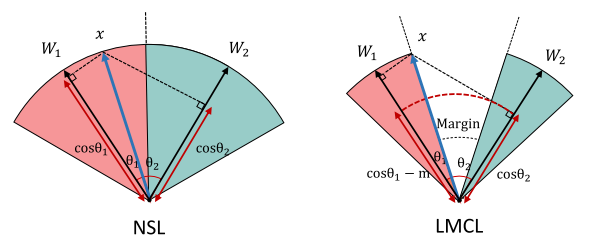## ArcFace

ArcFace: Additive Angular Margin Loss for Deep Face Recognition

$L_{3}=-\frac{1}{N} \sum_{i=1}^{N} \log \frac{e^{s\left(\cos \left(\theta_{y_{i}}+m\right)\right)}}{e^{s\left(\cos \left(\theta_{y_{i}}+m\right)\right)}+\sum_{j=1, j \neq y_{i}}^{n} e^{s \cos \theta_{j}}}$

$\\cos \left(\theta_{y_{i}}+m\right)$相当于原始的余弦函数在x轴上向左偏移。论文中将余弦余量$m$设置为 0.35(AM-Softmax, CosFace)。与 SphereFace 相比，附加余弦余量（CosineFace）有三个优点：

• 非常容易实现，没有棘手的超参数;
• 没有Softmax监督，更清晰，更易收敛;
• 性能有明显改善。## Summary

L-Softmax$\cos m\theta_{y_{i}}$）、A-Softmax$\cos m\theta_{y_{i}}$）、AM-Softmax$\cos\theta_{y_{i}}-m$）、CosFace$\cos\theta_{y_{i}}-m$）、ArcFace$\\cos \left(\theta_{y_{i}}+m\right)$）的本质都是对cosine函数以及margin形式进行改进。区别在于不同的函数形式在前向传播和反向传播时所需的计算量有所不同。

cosine函数的图像为：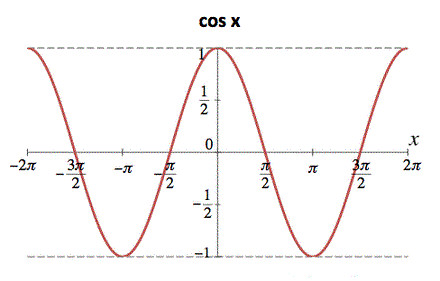## Blogs

posted @ 2019-03-15 16:11  天涯惟笑  阅读(1949)  评论(0编辑  收藏  举报